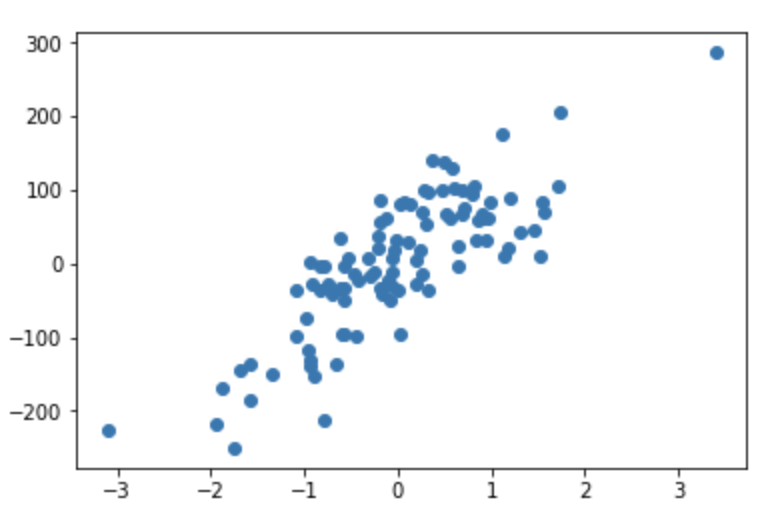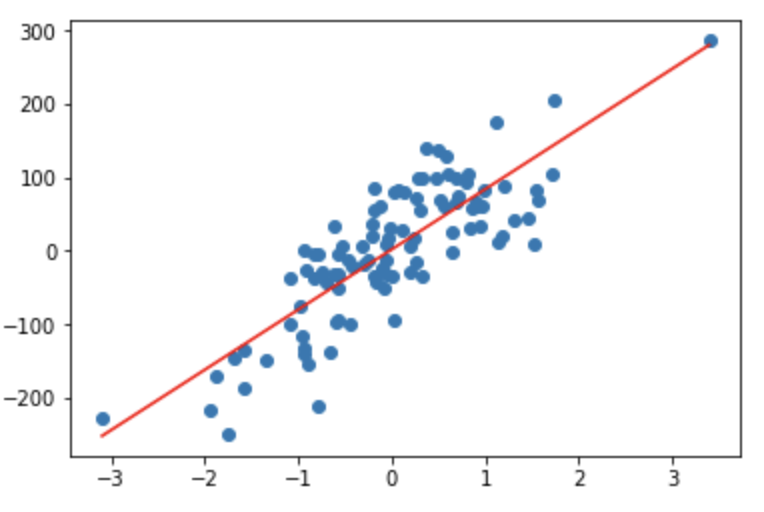# Linear Regression Using Gradient Descent[math]

27 Feb 2020

## What is Linear Regression?

In simplest terms, Linear Regression can be explained as just the curve fitting (straight line in this case) to the given set of data points. Linear Regression solves the following question: "Given a set of data points, what is the slope and y-intercept of the straight line which fits the data points perfectly?"

If you remember the elementry maths you would surely know the formula of a straight line, which is $y = mx + c$. Where, $m$ is the slope and $c$ is the y-intercept of the line!

Now when I say "the line which fits perfectly", that means the line placed in such a way that for any input $x$, it minimizes the difference between actual value (label: $y$) and the predected value $\bar{y}$. In our case, the predicted value is the point on the line at given $x$.

This cost function can be given by following equation and is called "Mean Squared Error" (Here I use $\theta_0$ and $\theta_1$ instead of $m$ and $c$ for slope and y-intercept respectively.) :

$$E = \frac{1}{n}\sum_{i=0}^{n} (y_i - \bar{y}_i)^2$$

Where $y$ is actual value and $\bar{y}$ is predicted value.

Our goal is to minimize the mean squared error (loss or cost). This can be iteratively done using Gradient descent. Here's the approach:

$$\theta := \theta - \alpha \frac{\partial E}{\partial \theta}$$

Intuition behind this equation is that gradient of curve at any point gives the direction of steepest ascent. But we are minimizing the error, So we need steepest descent and that's why there is -ve sign (Opposite of steepest ascent direction) in above equation.

Here, in above equation, $\theta$ is a vector with value:

$$\begin{bmatrix} \theta_0 \\ \theta_1 \end{bmatrix}$$ $\alpha$ is step size or learning rate and $\frac{\partial E}{\partial \theta}$ is derivative of MSE equation with respect to both $\theta_0$ and $\theta_1$. So $$\frac{\partial E}{\partial \theta} = \begin{bmatrix} \frac{\partial E}{\partial \theta_0} \\ \frac{\partial E}{\partial \theta_1} \end{bmatrix}$$

Now, let's substitute the value of $\bar{y}$ in equation of MSE:

\begin{align} \\ E & = \frac{1}{n}\sum_{i=0}^{n} (y_i - (\theta_0 + \theta_1X))^2 \\ \therefore \frac{\partial E}{\partial \theta_0} & = -\frac{2}{n}\sum_{i=0}^{n} (y_i - (\theta_0 + \theta_1X)) \\ & = -\frac{2}{n}\sum_{i=0}^{n} (y_i - \bar{y}) \\ \end{align} and \begin{align} \frac{\partial E}{\partial \theta_1} & = -\frac{2}{n}\sum_{i=0}^{n} X (y_i - (\theta_0 + \theta_1X)) \\ & = -\frac{2}{n}\sum_{i=0}^{n}X (y_i - \bar{y}) \\ \end{align}

Now Let's implement this concept (We won't implement the vectorized version as explained above; as we will work with only 1 feature)

Let's first import required libraries:

Now, for this tutorial purpose we won't use the actual dataset to train on, but instead we will generate the dataset on the fly as following:

You can scatter plot the data generated by above function using following code:

Which should look similar to following plot:Let's define some constants like learning rate $(\alpha)$

Initially we don't know anything about $\theta_0$ and $\theta_1$. So we'll initialize both with 0 and start improving using gradient descent algorithm.

Let's create some helper function for calculating MSE and for making predictions.

Finally the gradient descent loop, in which I'll use the same equations explained above:

The above training loop ends when the difference between consicutive errors is smaller than the $TOLERANCE$ defined in constants. It also prints the learned parameters.

You can finally plot the dataset and the resultant best fit line using following code:

Which should look similar to following plot: